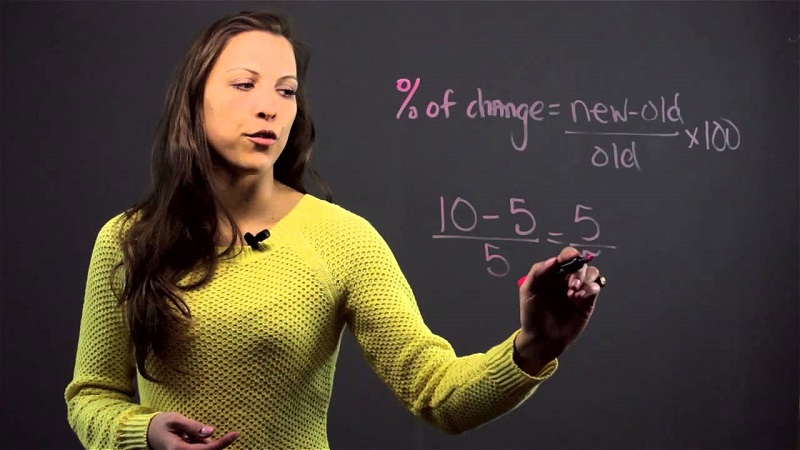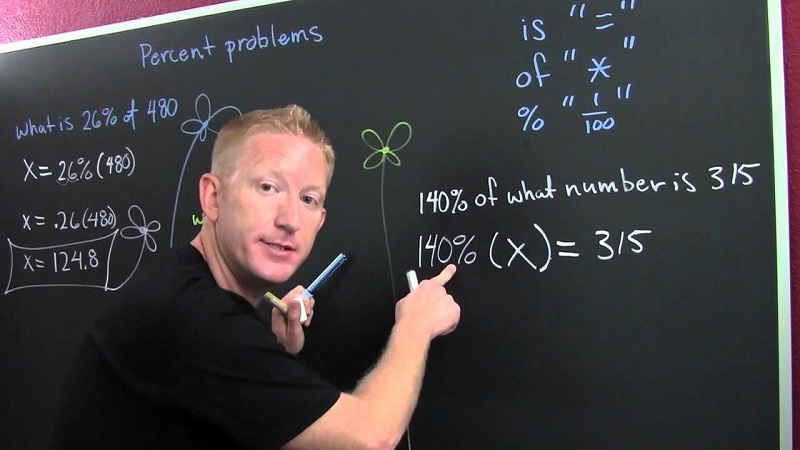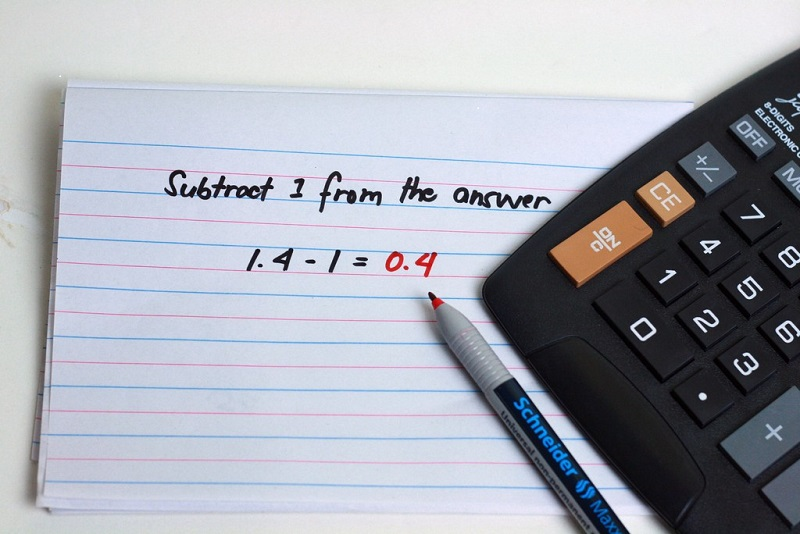# How to Find Percentage of a Number Very Easily?

Affiliate disclosure: In full transparency – some of the links on our website are affiliate links, if you use them to make a purchase we will earn a commission at no additional cost for you (none whatsoever!).

Are you finding it difficult to calculate percentage of a number? Don’t worry we are here to figure it all.

Our very first step would be to know what percentage means. The term “percent” in English comes from the Italian per cento or the French pour cent, which literally mean per hundred. We all know in mathematics that a percentage is a number or ratio expressed as a fraction of 100. It is often denoted using the percent sign “%“.

In this article, we will tell you How to Find Percentage of a number Very Easily? So, let’s get started:

## How to Find Percentage of a number Very Easily?### Method 1: Understanding Whole and Solving it Manually

Step #1: Taking an example would be a better way to show how to calculate percentage. So for an instance if there are 10 boxes then it means 100% as a whole. What this whole means now, the answer is that whole is just the way to express a number for percentage. Now if we remove 5 boxes, then we would have ((5/10)*100) %=50% of the boxes removed and 50% of the boxes left.

Step #2: You might be confused what this whole has to do with percentage so to find out whole let us put 10 red balls and 10 blue balls in a box. This will make a total of 20 balls in that box. This leads to a box with 20 balls as a whole which makes it equal to 100% of the box.

Step #3: Now if we need to figure out how to calculate the percentage of the box that is taken by blue balls kept in the box.

For this, we need to know how many blue balls are there in the box which is 10 blue balls, and the total number of balls in that box are 20. Now we will fraction these numbers that is 10 (total blue balls in the box)/20(total no. of balls kept in the box).

Step #4: To know how to find a percentage we will divide 10/20 and then make it into decimal form. This results out to be .5 (point 5).

Step #5: Finally we will get to know how to find the percentage of a number by multiplying the result by 100 to get the required percentage. Simply move the decimal to the right two places. In this case, the result would be 50% which is indeed true to the obvious reasons as 10 out of 20 balls makes the 50% of the whole.

Let us do a quick recap of all the processes and steps for finding percentages easily which we have discussed till this time.

If you are to calculate the percentage of any number then all that you have to do is to make the desired number “Dividend” of which you have to find the percentage and make another number “Divisor” which is the whole. Once you have got the result by performing the division then as the last step, you require multiplying the result by 100, and this will give you the percentage of that desired number.

Are you struggling with your writing skills? Here are something that can help you in improving your Writing skills. Do check that out.

### Method 2: Making use of online web-based applications.If you are looking for the easiest way to find percentages, then this will be our say. By making use of this method, you will be able to find percentages of any number easily. So, let’s get started with the method:

If you will make a search by the keyword “percentage calculator online” believe us, you will find a plethora of them. You can make use of either one of them but for the sake of ease and convenience, we will be showing you how to use one of them.

In this process, we will be continuing our journey with such an online web-based platform which is named as www.percentagecalculator.net.

You can do a lot of complex mathematical calculations on Microsoft Excel, here is an easy guide about calculating Average in MS Excel.

Step #1: As the very first step of this method, you will need to visit www.percentagecalculator.net. Once you have done the mentioned, then you are all set to go to the next step.

Step #2: Now, you will be seeing 3 different sections. Let us explain the use of each one of them with the help of an example.

For instance, if you are to figures out that what is 50 % of 100 then the very first section of percentage calculator is for you.

All what you have to do is to enter the percentage at the first field and the total quantity in the second field. Now, as the final thing, you will require clicking on calculate and the field next to calculate will tell you the answer.

Step #3: The second section will help you to figure out that what is the percentage of a certain portion out of whole. Let us explain it using the example as well.

For instance, if you are to calculate that 50 is what percent of 100 then this section is for you. You just have to enter the numerals at the corresponding field and after clicking on calculate, the result will be in front of you.

Step #4: The last section is of some great use. It can be used if you are to calculate that to what number the percentage will increase or decrease if you provide the numbers.

Like, for instance, if you have to find that what is the percentage increase from 10 to 20? Then this section will do the required for you. Obviously, the result will come out to be 100% once you hit enter.### Method 3: Making use of calculators.

Alternatively, you can consider using manual calculators for your use. Nowadays, every smartphone is having a calculator in it. Additionally, other manual calculators are also available in the market which can be of great use if the first two methods don’t entertain you.

### Let’s wrap it up.

This lets’s wrap it up section brings us to the end of this tutorial on “How to find percentage?” We hope that we were efficient enough to satisfy your curiosity about this topic. Here is something related that can help you a lot. See how to add numbers in Excel. Using MS Excel, you can add a whole lot of data in few seconds.

We firmly believe that we have left no stone unturned while getting into the nitty gritty of the topic and making you understand it. But if you still have any query regarding how to do percentages? Then feel free to drop your query via comments below. We will try our level best to resolve that.##### arun singh

Arun Singh is a experienced server management geek and he has more than 8 years of experienced handling hosting server and he do sometimes share his knowledge in digital marketing. He currently with private company in Mumbai, India and he handles server management at BloggersIdeas.com.